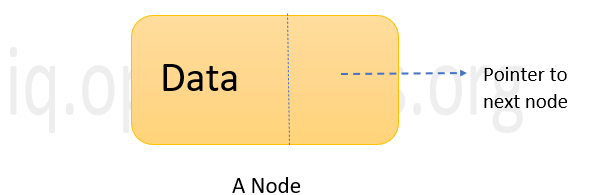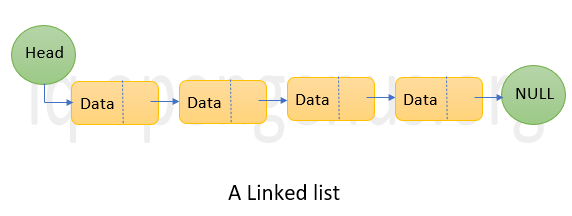×

Search anything:

#### Algorithms List of Mathematical Algorithms linked listGet this book -> Problems on Array: For Interviews and Competitive Programming

In this article, we will represent an Integer as Singly Linked List and define an addition algorithm to add two integers as Linked Lists. This is used to add very large integers which is not stored in existing data types.

• Algorithm
• Representing a number as a linked lists
• Implementation
• Example
• Time and space complexity

Linked lists are a type of data structure that stores data in a dynamically allocated memory space. The data is stored in nodes. Each node has a pointer or reference link that points to the next node. So, a node consists of two components: one to store the required data and another to point to the next node. Hence we can come to a conclusion that linked lists are a collection of nodes. The pointer of the last node always points to 'NULL' indicating the end of the list.The above represented linked list is called a Singly Linked list as a given node has a pointer that points to only one other node i.e. the next node. If a given node of a linked list points to two other nodes i.e.both the previous node and the next node, such a list is called Doubly linked list.

For adding two integers as linked lists, the approach used here is given below.

## Algorithm

• Get the two numbers to be added from the user.
• Create two linked lists where the digits of each number are pushed in a reverse manner.
• Result is then stored in another linked list which is then reversed
• Display the Result.

Here, the digits of the numbers are stored in the linked list in reverse order so that addition operation can start from the least significant bit of the numbers. We have made use of the singly linked list here.

## Implementation

The below given is the python code to perform this operation.

### Initialization

``````import math

class node:
def __init__(self,value):
self.value=value
self.next=None
class LList:
def __init__(self):
``````

For execution, two classes are defined:

• a class node
• a class LList

In the class node, the pointers characteristic to the node like self.value and self.next are initialized as the value that is given to be stored in that node and None respectively. The pointer self.value is used to point to the value or data stored in that particular node and the pointer self.next is used to point to the next corresponding node in the linked list and store its address. The following functions are defined under the class LList:

• __ init __(self): This is similar to a constructor that initializes the pointer self.head as None, which is used to point the head node or the starting node of the linked list.

## Represent Numbers as Linked Lists

``````def insertnumber(self):
number=int(input("Enter the number: "))
temp=number

# to create linked list in reverse
power = 1
while( power * 10 < temp):
power = power * 10;
while temp>0:
#get inidividual digits of the number and push it into the linked list
digit = math.floor(temp / power)
self.push(digit)
temp = temp % power
power = power / 10
``````
• insertnumber (self): This function gets the number from the user and pushes each digit into the linked list as follows:
1. The input is got from the user.
2. Two variables temp and power are initialized where temp=number and power represents the power of 10 < number.
3. The variable temp is divided by power and the result is pushed into the linked ist using push function.
4. The values of temp and power are updated as temp%10 and power/10 respectively.
5. Steps 1-4 are repeated until temp is a non-zero integer.

For example, lets take the number to be 123.
Initially, power=10, temp=23

Iteration 1: Digit=23/10=1   (2 is pushed into the linked list)
Then, temp and power are updated as 3 and 1
respectively.

Iteration 2: Digit=3/1=3   (3 is pushed into the linked list)
Then, temp and power are updated as 0 and 1
respectively

Since temp=0, the iteration is terminated.

### Pushing the number into linked list

``````def push(self,data):
new_node = node(data)
``````
• push (self, data): This function gets the digit to be pushed into the linked list and it performs this in a manner such that the digits are pushed into the linked list in the reverse order.

Let's continue with our previous example. Say, 2 and 3 are pushed into the linked list. First, a new node is created with 2 as its data. Then, another node is inserted befor this node and 3 is inserted. So the linked list looks like 3 -> 2

`````` def add(self,list1,list2):
carry=0
prev=None
temp=None
Sum=0
while(list1 is not None or list2 is not None):
#If either of the two numbers are short, 0 is taken as the value for the shorter number's digit;
#else the respective digits of the two numbers are added
l1_data=0 if list1 is None else list1.value
l2_data=0 if list2 is None else list2.value
Sum=l1_data+l2_data+carry

# to re-initialize the values of sum and carry
if Sum<10:
carry = 0
Sum=Sum
else:
carry = 1
Sum=Sum%10
#create a new node to store the Sum value
temp=node(Sum)
else:
prev.next = temp
prev = temp
#moving the pointer to the next digit
if list1 is not None:
list1=list1.next
if list2 is not None:
list2=list2.next
``````
• add (self, list1, list2): This function is used to add the two numbers digit by digit. Since the numbers are stored in reverse manner in the linked lists, the resultant linked list will also contain the answer in the reverse manner.It is done as follows:
1. The head node of both the linked lists are passed as inputs of the function.
2. The variables temp, Sum, carry, and prev are initialized.
3. The variables l1_data and l2_data are to store the value of that particular node of both lists. If one list is shorter than the other, its data is stored as 0.
4. Addition is performed as Sum=l1_data+l2_data+carry.
5. The values of Sum and carry are re-initialized as carry=0 and Sum=Sum if Sum<10 else, carry=1 and Sum=Sum%10.
6. A new temporary linked list is created to store the result.
7. The pointers of the two linked lists are moved to the next node to perform the addition.
8. Steps 1-7 are repeated until nodes of both lists have been traversed.

## Reverse the resultant linked list

``````def reverse(self):
prev = None
while(current is not None):
next = current.next
current.next = prev
prev = current
current = next
``````
• reverse (self): This function is used to reverse the linked list. This is carried out on the resultant linked list so that the result could be displayed in the proper order of digits.

## Printing the result

``````#to print the result
def printList(self):
while(temp):
print(temp.value, end=" "),
temp = temp.next
``````
• printList (self): This function prints the linked list.

### Driver code

``````num1=LList() #creating a linked list to store the addend
num2=LList() #creating a linked list to store the augend
result=LList() # A linked list to store the result
num1.insertnumber()
num2.insertnumber()
result.reverse()
result.printList()
``````
1. Three linked lists are created to store the addend, augend and the result.
2. Then the insertnumber() method is called on the linked lists num1 and num2 to get the numbers and store them in linked lists.
3. The add() function is called on the result linked list and the heads of num1 and num2 are passed as arguments.
4. the reverse() is called on result linked list to reverse the linked list and then printList() is called to print the result.

## Example

Let the inputs be 1234 and 1.

#### Input

``````Enter the number: 1234
Enter the number:    1
``````

The linked lists will be :
4 -> 3 -> 2 -> 1
1

4 and 1 are the head nodes of both the linked lists.The addition will take place as follows:

``````Sum=0, carry=0, prev=None, temp=None
``````

Iteration 1:

```l1_data=4 , l2_data=1
Sum=4+1+0
Sum=5 , carry=0
temp= 5```

Iteration 2:
```l1_data=3 , l2_data=0
Sum=3+0+0
Sum=3 , carry=0
temp= 5 -> 3```

Iteration 3:
```l1_data=2 , l2_data=0
Sum=2+0+0
Sum=2 , carry=0
temp= 5 -> 3 -> 2```

Iteration 4:
```l1_data=1 , l2_data=0
Sum=1+0+0
Sum=1 , carry=0
temp= 5 -> 3 -> 2 -> 1```

After iteration 4, the nodes of both the linked lists have been traversed. So the iteration is stopped and the result is 5 -> 3 -> 2 -> 1.

The linked list result is then reversed to give 1 -> 2 -> 3 -> 5 and it is then printed.

#### Output

``````1235
``````

# Time and space Complexity

Let m and n be the number of nodes in the linked lists of addend and augend respectively. Since each node is traversed only once, the time complexity is O(M+N).

The space complexity is also O(M+N) as the memory is occupied by the two linked lists only and the result is stored in a temporary linked list.

By the end of this article at OpenGenus, you will have a clear understanding on what are linked lists and how to add two integers that are represented by linked lists.#### Sanjana Babu

Sanjana Babu is a Student at SRM Valliammai Engineering College and is an Intern at OpenGenus. She is a Philomath.